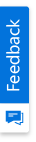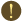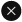We use cookies to give you the best experience on our website. If you continue to browse, then you agree to our privacy policy and cookie policy.Unfortunately, activation email could not send to your email. Please try again.Read Excel file in C#, VB.NET

Platform: WinForms |
Control: XlsIO |
Published Date: August 6, 2018 |
Last Revised Date: December 4, 2019
Tags:

Read Excel file and write into Excel file are the common features that are very essential for almost every business application.

The Syncfusion Excel (XlsIO) library is a .NET Excel library used to create, read, and edit Excel documents without the dependency of interop Excel library. Also, converts Excel documents to PDF files and much more.

This article demonstrates how to read Excel file and Excel data in C# and VB.NET by using Syncfusion Excel (XlsIO) library. The code examples for the following operations are also shown.

• Read/Write an Excel file and read different types of Excel data
• Iterate the cells in the used range of the Excel file to read Excel data
• Read an Excel file and export to DataTable

Steps to read Excel file and Excel data programmatically:

Step 1: Create a new C# console application project.Create a new C# console application

Step 2: Install Syncfusion.XlsIO.WinForms NuGet package as a reference to your .NET Framework applications from the NuGet.org.Install XlsIO NuGet package

Step 3: Include the following namespace in the Program.cs file.

C#

using Syncfusion.XlsIO;

VB.NET

Imports Syncfusion.XlsIO

Step 4: Add the below code snippets to read Excel file and its data. The description of some important class members used in the code examples are as follows:

• ExcelEngine should be initialized to access the Excel object.
• The Open() method of IWorkbooks interface opens the existing Excel workbook from the specified location. Moreover, you can also open the specified stream.
• You can access any of the Worksheets with its zero-based index for further manipulations.
• String values can be read from a cell range using the Text property.
• Likewise, number values can be read from a cell range using the Number property.
• Date and time values can be read from a cell using the DateTime property.
• Excel formulas can be read using the Formula property.
• The EnableSheetCalculations() method of IWorksheet interface should be invoked to perform calculation in an Excel workbook, and to evaluate formula.
• Excel formulas can be evaluated using the CalculatedValue property.
• The DisplayText property can be used to retrieve the resultant value of a cell with its number format applied.
• Using the UsedRange property, you can get the used range on the specified worksheet.
• Finally, the SaveAs() method can be used to save the Excel file to the disk.
• The ExportDataTable() method can be used to export worksheet data in the specified range into a DataTable.

Read Excel file and the different types of Excel data

The following code snippet in C# and VB.NET reads an Excel file, prints the different types of data present in the Excel file, and writes the loaded Excel file.

C#

using (ExcelEngine excelEngine = new ExcelEngine())
{
//Initialize application
IApplication app = excelEngine.Excel;

//Set default application version as Excel 2016
app.DefaultVersion = ExcelVersion.Excel2016;

//Open existing Excel workbook from the specified location
string inputFileName = "Sample.xlsx";
IWorkbook workbook = app.Workbooks.Open(inputFileName, ExcelOpenType.Automatic);

//Access the first worksheet
IWorksheet worksheet = workbook.Worksheets;

int id = (int)worksheet["A2"].Number;

string name = worksheet["B2"].Text;

DateTime dob = worksheet["C2"].DateTime;

string ageFormula = worksheet["D2"].Formula;

//Enable calculations
worksheet.EnableSheetCalculations();

string formulaValue = worksheet["D2"].CalculatedValue;

//Get display text
string displayText = worksheet["C2"].DisplayText;

//Save the Excel workbook to disk in xlsx format
workbook.SaveAs("Output.xlsx");

//Print the values that are read from the Excel file
Console.WriteLine("Number in cell A2: {0}", id);
Console.WriteLine("Text in cell B2: {0}", name);
Console.WriteLine("DateTime in cell C2: {0}", dob);
Console.WriteLine("Formula in cell D2: {0}", ageFormula);
Console.WriteLine("Calculated formula value in cell D2: {0}", formulaValue);
Console.WriteLine("Display text in cell C2: {0}", displayText);
}

VB.NET

Using excelEngine As ExcelEngine = New ExcelEngine

'Initialize application
Dim app As IApplication = excelEngine.Excel

'Set default application version as Excel 2016
app.DefaultVersion = ExcelVersion.Excel2016

'Open existing Excel workbook from the specified location
Dim inputFileName As String = "Sample.xlsx"
Dim workbook As IWorkbook = app.Workbooks.Open(inputFileName, ExcelOpenType.Automatic)

'Access the first worksheet
Dim worksheet As IWorksheet = workbook.Worksheets(0)

Dim id As Integer = CInt(worksheet("A2").Number)

Dim name As String = worksheet("B2").Text

Dim dob As DateTime = worksheet("C2").DateTime

Dim ageFormula As String = worksheet("D2").Formula

'Enable calculations
worksheet.EnableSheetCalculations()

Dim formulaValue As String = worksheet("D2").CalculatedValue

'Get display text
Dim displayText As String = worksheet("C2").DisplayText

'Save the Excel workbook to disk in xlsx format
workbook.SaveAs("Output.xlsx")

'Print the values that are read from the Excel file
Console.WriteLine("Number in cell A2: {0}", id)
Console.WriteLine("Text in cell B2: {0}", name)
Console.WriteLine("DateTime in cell C2: {0}", dob)
Console.WriteLine("Formula in cell D2: {0}", ageFormula)
Console.WriteLine("Calculated formula value in cell D2: {0}", formulaValue)
Console.WriteLine("Display text in cell C2: {0}", displayText)
End Using

A complete working example to read Excel file and its data from code along with input Excel file used can be downloaded from Read Excel File.zip.

By executing the program, you will get the loaded and saved Excel file as shown below.Also, the values are read from the Excel file and printed in the output console window as follows.Read Excel data in console output window

Read Excel data within the used range – cell by cell iteration

The following code snippet reads data within the used range of the Excel file by iterating rows and columns.

C#

using (ExcelEngine excelEngine = new ExcelEngine())
{
//Initialize application
IApplication app = excelEngine.Excel;

//Set default application version as Excel 2016
app.DefaultVersion = ExcelVersion.Excel2016;

//Open existing Excel workbook from the specified location
string inputFileName = "Sample.xlsx";
IWorkbook workbook = app.Workbooks.Open(inputFileName, ExcelOpenType.Automatic);

//Access the first worksheet
IWorksheet worksheet = workbook.Worksheets;

//Access the used range of the Excel file
IRange usedRange = worksheet.UsedRange;
int lastRow = usedRange.LastRow;
int lastColumn = usedRange.LastColumn;
//Iterate the cells in the used range and print the cell values
for (int row = 1; row <= lastRow; row++)
{
for (int col = 1; col <= lastColumn; col++)
{
Console.Write(worksheet[row, col].Value);
Console.Write("\t\t");
}
Console.WriteLine("\n");
}
Console.WriteLine("\n\n");
//Iterate the cells in the used range and print the display text
for (int row = 1; row <= lastRow; row++)
{
for (int col = 1; col <= lastColumn; col++)
{
Console.Write(worksheet[row, col].DisplayText);
Console.Write("\t\t");
}
Console.WriteLine("\n");
}
}

VB.NET

Using excelEngine As ExcelEngine = New ExcelEngine

'Initialize application
Dim app As IApplication = excelEngine.Excel

'Set default application version as Excel 2016
app.DefaultVersion = ExcelVersion.Excel2016

'Open existing Excel workbook from the specified location
Dim inputFileName As String = "Sample.xlsx"
Dim workbook As IWorkbook = app.Workbooks.Open(inputFileName, ExcelOpenType.Automatic)

'Access the first worksheet
Dim worksheet As IWorksheet = workbook.Worksheets(0)

'Access the used range of the Excel file
Dim usedRange As IRange = worksheet.UsedRange
Dim lastRow As Integer = usedRange.LastRow
Dim lastColumn As Integer = usedRange.LastColumn
'Iterate the cells in the used range and print the cell values
For row As Integer = 1 To lastRow
For col As Integer = 1 To lastColumn
Console.Write(worksheet(row, col).Value)
Console.Write(vbTab & vbTab)
Next
Console.WriteLine(vbLf)
Next
Console.WriteLine(vbLf & vbLf)
'Iterate the cells in the used range and print the display text
For row As Integer = 1 To lastRow
For col As Integer = 1 To lastColumn
Console.Write(worksheet(row, col).DisplayText)
Console.Write(vbTab & vbTab)
Next
Console.WriteLine(vbLf)
Next
End Using

A complete working example to iterate each cell in the used range and read data from an Excel file in C# and VB.NET can be downloaded from Read Excel data in used range.zip.

The following is the screenshot of the sample Excel file.Sample input Excel document

By executing the program, you will get the following output.Cell values and display text in the used range

Read Excel file and export to DataTable in C# and VB.NET

To use the DataTable object, the following namespace must be included in addition to `Syncfusion.XlsIO`.

C#

using System.Data;

VB.NET

Imports System.Data

Now, the following code example helps you to read an Excel file and load it to DataTable.

C#

using (ExcelEngine excelEngine = new ExcelEngine())
{
//Initialize application
IApplication app = excelEngine.Excel;

//Set default application version as Excel 2016
app.DefaultVersion = ExcelVersion.Excel2016;

//Open existing Excel workbook from the specified location
string inputFileName = "Sample.xlsx";
IWorkbook workbook = app.Workbooks.Open(inputFileName, ExcelOpenType.Automatic);

//Access the first worksheet
IWorksheet worksheet = workbook.Worksheets;

//Export Excel to DataTable
DataTable dataTable = worksheet.ExportDataTable(worksheet.UsedRange, ExcelExportDataTableOptions.ColumnNames);
}

VB.NET

Using excelEngine As ExcelEngine = New ExcelEngine

'Initialize application
Dim app As IApplication = excelEngine.Excel

'Set default application version as Excel 2016
app.DefaultVersion = ExcelVersion.Excel2016

'Open existing Excel workbook from the specified location
Dim inputFileName As String = "Sample.xlsx"
Dim workbook As IWorkbook = app.Workbooks.Open(inputFileName, ExcelOpenType.Automatic)

'Access the first worksheet
Dim worksheet As IWorksheet = workbook.Worksheets(0)

'Export Excel to DataTable
Dim dataTable As DataTable = worksheet.ExportDataTable(worksheet.UsedRange, ExcelExportDataTableOptions.ColumnNames)
End Using

A complete working example to read an Excel file and export it to DataTable in C# and VB.NET can be downloaded from Excel to DataTable.zip.

By executing the program, you will get the DataTable as follows.DataTable exported from Excel file

Take a moment to explore the rich set of Syncfusion Excel (XlsIO) library features.

To learn more about the Syncfusion Excel (XlsIO) library, refer to the documentation where you will find basic worksheet data manipulation options along with features like Conditional Formatting, worksheet calculations through Formulas, adding Charts in worksheet or workbook, organizing and analyzing data through Tables and Pivot Tables, appending multiple records to worksheet using Template Markers, and most importantly PDF and Image conversions with code examples.

Create an Excel file in C# and VB.NET

Convert Excel file to CSV in C# and VB.NET

Insert the Excel rows and columns in C# and VB.NET

Copy an Excel worksheet to another workbook in C# and VB.NET

Merge or combine multiple Excel files in C# and VB.NET

Create an Excel file in ASP.NET Core

Create an Excel file in ASP.NET MVC

Create an Excel file in ASP.NET Web Forms

Create an Excel file in WPF

Create an Excel file in UWP

Create an Excel file in Xamarin

Create an Excel file in Xamarin.Android

Create an Excel file in Xamarin.iOS

Create an Excel file in Azure platform

Note:

Starting with v16.2.0.x, if you reference Syncfusion assemblies from trial setup or from the NuGet feed, include a license key in your projects. Refer to the link to learn about generating and registering Syncfusion license key in your application to use the components without trial message.

2X faster development

The ultimate WinForms UI toolkit to boost your development speed.You are using an outdated version of Internet Explorer that may not display all features of this and other websites. Upgrade to Internet Explorer 8 or newer for a better experience.